ISEE Middle Level Quantitative : How to multiply variables

Example Questions

1 2 3 4 5 6 7 8 10 Next →

Example Question #121 : Variables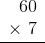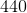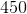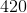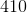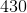Explanation:

To solve this problem, we multiplyby the ones position and the tens position.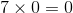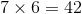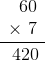Example Question #122 : Variables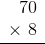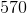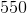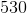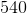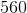Explanation:

To solve this problem, we multiplyby the ones position and the tens position.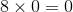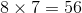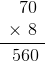Example Question #123 : Variables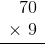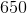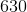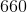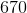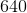Explanation:

To solve this problem, we multiplyby the ones position and the tens position.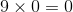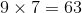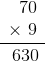Example Question #121 : Variables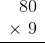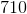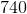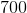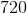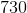Explanation:

To solve this problem, we multiplyby the ones position and the tens position.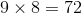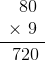Example Question #125 : Variables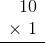Explanation:

To solve this problem, we multiplyby the ones position and the tens position.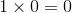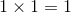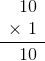Example Question #126 : Variables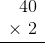Explanation:

To solve this problem, we multiplyby the ones position and the tens position.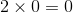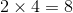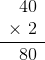Example Question #127 : Variables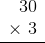Explanation:

To solve this problem, we multiplyby the ones position and the tens position.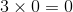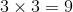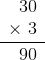1 2 3 4 5 6 7 8 10 Next →